Tweeter Mids Woofer Passive Radiators no longer sold

# SB Acoustics

## Tweeter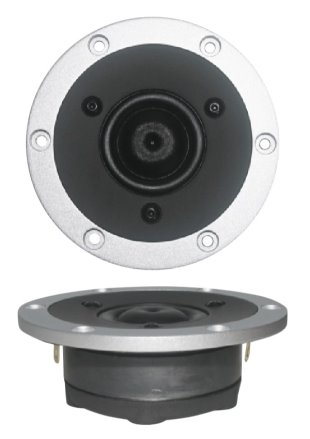open a bigger photo

## SB Acoustics Satori TW29R

order no. sb-SATORI-TW29R

EUR 134,00excl. VAT: € 112.61 / \$ 124.99

.

• power handling (continuous/programme) = 100 W
• frequency range = 400-35000 Hz
• resonance frequency fs = 600 Hz
• impedance R = 4 Ohm
• sound pressure level SPL = 92,5 dB (2,83V; 1m)
• DC resistance Re = 3 Ohm
• force factor BL = 2 N/A
• voice coil inductance L = 0,02 mH
• effective piston radiating area Sd = 9,6 cm2
• effective mechanical mass incl. air load mms = 0,45 g
• total Q factor Qts = 0,76 (Qms=2, Qes=1,22)
• voice coil diameter = 29 mm
• mounting diameter d = 70 mm
• overall diameter d = 103,8 mm
• mounting depth (not countersunk) t = 37,25 mm
PDF data sheetopen a bigger photo

EUR 45,10excl. VAT: € 37.90 / \$ 42.07

.

• power handling (continuous/programme) = 120 W
• frequency range = 400-42000 Hz
• resonance frequency fs = 680 Hz
• impedance R = 4 Ohm
• sound pressure level SPL = 90 dB (2,83V; 1m)
• DC resistance Re = 3,2 Ohm
• force factor BL = 1,6 N/A
• voice coil inductance L = 0,04 mH
• effective piston radiating area Sd = 6,2 cm2
• effective mechanical mass incl. air load mms = 0,38 g
• total Q factor Qts = 1,11 (Qms=2,7, Qes=1,88)
• voice coil diameter = 25,4 mm
• mounting diameter d = 82,8 mm
• overall diameter d = 100 mm
• mounting depth (not countersunk) t = 38,63 mm
PDF data sheet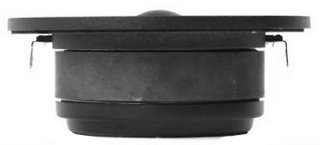open a bigger photo

## SB Acoustics SB26STAC-C000-4

order no. sb-26STAC-C000-4

EUR 36,90excl. VAT: € 31.01 / \$ 34.42

.

• power handling (continuous/programme) = 120 W
• frequency range = 500-40000 Hz
• resonance frequency fs = 750 Hz
• impedance R = 4 Ohm
• sound pressure level SPL = 91,5 dB (2,83V; 1m)
• DC resistance Re = 3,2 Ohm
• force factor BL = 1,6 N/A
• voice coil inductance L = 0,04 mH
• effective piston radiating area Sd = 6,2 cm2
• effective mechanical mass incl. air load mms = 0,3 g
• total Q factor Qts = 1,12 (Qms=3, Qes=1,78)
• voice coil diameter = 25,4 mm
• mounting diameter d = 82,8 mm
• overall diameter d = 100 mm
• mounting depth (not countersunk) t = 39,63 mm
PDF data sheetopen a bigger photo

## SB Acoustics SB26STC-C000-4

order no. sb-26STC-C000-4

EUR 26,70excl. VAT: € 22.44 / \$ 24.91

.

• power handling (continuous/programme) = 120 W
• frequency range = 400-40000 Hz
• resonance frequency fs = 750 Hz
• impedance R = 4 Ohm
• sound pressure level SPL = 91,5 dB (2,83V; 1m)
• DC resistance Re = 3,2 Ohm
• force factor BL = 1,6 N/A
• voice coil inductance L = 0,04 mH
• effective piston radiating area Sd = 6,2 cm2
• effective mechanical mass incl. air load mms = 0,3 g
• total Q factor Qts = 1,12 (Qms=3, Qes=1,78)
• voice coil diameter = 25,4 mm
• mounting diameter d = 82,8 mm
• overall diameter d = 100 mm
• mounting depth (not countersunk) t = 39,63 mm
PDF data sheetopen a bigger photo

## SB Acoustics SB26STCN-C000-4

order no. sb-26STCN-C000-4

EUR 28,70excl. VAT: € 24.12 / \$ 26.77

.

• power handling (continuous/programme) = 120 W
• frequency range = 600-35000 Hz
• resonance frequency fs = 960 Hz
• impedance R = 4 Ohm
• sound pressure level SPL = 92,5 dB (2,83V; 1m)
• DC resistance Re = 3,2 Ohm
• force factor BL = 1,8 N/A
• voice coil inductance L = 0,04 mH
• effective piston radiating area Sd = 6,2 cm2
• effective mechanical mass incl. air load mms = 0,3 g
• total Q factor Qts = 0,84 (Qms=1,6, Qes=1,77)
• voice coil diameter = 25,4 mm
• mounting diameter d = 46,15 mm
• overall diameter d = 72 mm
• mounting depth (not countersunk) t = 29,63 mm
PDF data sheetopen a bigger photo

## SB Acoustics SB29RDC-C000-4

order no. sb-29RDC-C000-4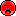- not available

.

• power handling (continuous/programme) = 100 W
• frequency range = 400-40000 Hz
• resonance frequency fs = 600 Hz
• impedance R = 4 Ohm
• sound pressure level SPL = 93 dB (2,83V; 1m)
• DC resistance Re = 3 Ohm
• force factor BL = 2,4 N/A
• voice coil inductance L = 0,05 mH
• effective piston radiating area Sd = 9,6 cm2
• effective mechanical mass incl. air load mms = 0,45 g
• total Q factor Qts = 0,64 (Qms=2,2, Qes=0,9)
• voice coil diameter = 29 mm
• mounting diameter d = 70 mm
• overall diameter d = 104 mm
• mounting depth (not countersunk) t = 37,55 mm
PDF data sheetopen a bigger photo

## SB Acoustics SB29RDNC-C000-4

order no. sb-29RDNC-C000-4

EUR 59,80excl. VAT: € 50.25 / \$ 55.78

.

• power handling (continuous/programme) = 100 W
• frequency range = 500-31000 Hz
• resonance frequency fs = 680 Hz
• impedance R = 4 Ohm
• sound pressure level SPL = 94 dB (2,83V; 1m)
• DC resistance Re = 3 Ohm
• force factor BL = 2,6 N/A
• voice coil inductance L = 0,05 mH
• effective piston radiating area Sd = 9,6 cm2
• effective mechanical mass incl. air load mms = 0,45 g
• total Q factor Qts = 0,45 (Qms=1,2, Qes=0,72)
• voice coil diameter = 29 mm
• mounting diameter d = 55,5 mm
• overall diameter d = 72 mm
• mounting depth (not countersunk) t = 30,63 mm
PDF data sheet

## Midsopen a bigger photo

recommended cabinet 1:
closed cabinet with 1,4 L volume
from 119/75 Hz (-3dB/-8dB)

recommended cabinet 2:
5,2 L volume bass reflex cabinet
with HP35 reflex tube, 11 cm long.
from 60/48 Hz (-3dB/-8dB).

## SB Acoustics 4” SB12MNRX25-4

order no. sb-12MNRX25-4

EUR 47,20excl. VAT: € 39.66 / \$ 44.03

.

• power handling (continuous/programme) = 50 W
• frequency range = 80-15000 Hz
• resonance frequency fs = 58 Hz
• impedance R = 4 Ohm
• sound pressure level SPL = 88,5 dB (2,83V; 1m)
• DC resistance Re = 3,2 Ohm
• force factor BL = 3,7 N/A
• voice coil inductance L = 0,14 mH
• effective piston radiating area Sd = 50 cm2
• effective mechanical mass incl. air load mms = 4,9 g
• equivalent volume of compliance Vas = 5,5 l
• total Q factor Qts = 0,38 (Qms=3,6, Qes=0,42)
• voice coil diameter = 25,4 mm
• mounting diameter d = 95 mm
• overall diameter d = 123 mm
• mounting depth (not countersunk) t = 69,61 mm
PDF data sheetopen a bigger photo

recommended cabinet 1:
closed cabinet with 2,1 L volume
from 99/62 Hz (-3dB/-8dB)

recommended cabinet 2:
8,7 L volume bass reflex cabinet
with HP35 reflex tube, 9 cm long.
from 46/39 Hz (-3dB/-8dB).

## SB Acoustics 4” SB12NRXF25-4

order no. sb-12NRXF25-4

EUR 49,20excl. VAT: € 41.34 / \$ 45.89

.

• power handling (continuous/programme) = 30 W
• frequency range = 80-12000 Hz
• resonance frequency fs = 61 Hz
• impedance R = 4 Ohm
• sound pressure level SPL = 87 dB (2,83V; 1m)
• DC resistance Re = 3,1 Ohm
• force factor BL = 3,6 N/A
• voice coil inductance L = 0,27 mH
• effective piston radiating area Sd = 50 cm2
• effective mechanical mass incl. air load mms = 5,8 g
• equivalent volume of compliance Vas = 4,1 l
• total Q factor Qts = 0,48 (Qms=5,3, Qes=0,53)
• voice coil diameter = 25,4 mm
• mounting diameter d = 95 mm
• overall diameter d = 123 mm
• mounting depth (not countersunk) t = 60,61 mm
PDF data sheetopen a bigger photo

recommended cabinet 1:
closed cabinet with 1,8 L volume
from 105/67 Hz (-3dB/-8dB)

recommended cabinet 2:
6,7 L volume bass reflex cabinet
with HP35 reflex tube, 11 cm long.
from 52/42 Hz (-3dB/-8dB).

## SB Acoustics 4” SB12NRX25-4

order no. sb-12NRX25-4

EUR 47,20excl. VAT: € 39.66 / \$ 44.03

.

• power handling (continuous/programme) = 30 W
• frequency range = 80-12000 Hz
• resonance frequency fs = 55 Hz
• impedance R = 4 Ohm
• sound pressure level SPL = 87,5 dB (2,83V; 1m)
• DC resistance Re = 3,1 Ohm
• force factor BL = 3,6 N/A
• voice coil inductance L = 0,14 mH
• effective piston radiating area Sd = 50 cm2
• effective mechanical mass incl. air load mms = 5,6 g
• equivalent volume of compliance Vas = 5,6 l
• total Q factor Qts = 0,4 (Qms=3,2, Qes=0,46)
• voice coil diameter = 25,4 mm
• mounting diameter d = 95 mm
• overall diameter d = 123 mm
• mounting depth (not countersunk) t = 60,61 mm
PDF data sheet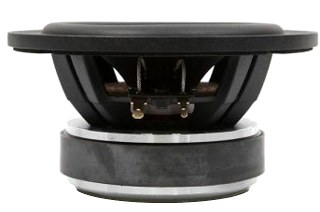open a bigger photo

recommended cabinet 1:
closed cabinet with 2,3 L volume
from 109/69 Hz (-3dB/-8dB)

recommended cabinet 2:
6,8 L volume bass reflex cabinet
with HP50 reflex tube, 23 cm long.
from 60/46 Hz (-3dB/-8dB).

## SB Acoustics 5” SB15NRXC30-4

order no. sb-15NRXC30-4

EUR 53,80excl. VAT: € 45.21 / \$ 50.18

.

• power handling (continuous/programme) = 50 W
• frequency range = 80-15000 Hz
• resonance frequency fs = 41 Hz
• impedance R = 4 Ohm
• sound pressure level SPL = 91 dB (2,83V; 1m)
• DC resistance Re = 3,1 Ohm
• force factor BL = 4,7 N/A
• voice coil inductance L = 0,12 mH
• effective piston radiating area Sd = 82 cm2
• effective mechanical mass incl. air load mms = 8,6 g
• equivalent volume of compliance Vas = 16,7 l
• total Q factor Qts = 0,29 (Qms=5,3, Qes=0,31)
• voice coil diameter = 30,5 mm
• mounting diameter d = 123 mm
• overall diameter d = 150 mm
• mounting depth (not countersunk) t = 69 mm
PDF data sheetopen a bigger photo

recommended cabinet 1:
closed cabinet with 3,5 L volume
from 92/58 Hz (-3dB/-8dB)

recommended cabinet 2:
11,4 L volume bass reflex cabinet
with HP50 reflex tube, 18 cm long.
from 49/38 Hz (-3dB/-8dB).

## SB Acoustics 5” SB15NRXC30-8

order no. sb-15NRXC30-8

EUR 53,80excl. VAT: € 45.21 / \$ 50.18

.

• power handling (continuous/programme) = 50 W
• frequency range = 80-15000 Hz
• resonance frequency fs = 38 Hz
• impedance R = 8 Ohm
• sound pressure level SPL = 88 dB (2,83V; 1m)
• DC resistance Re = 5,7 Ohm
• force factor BL = 5,6 N/A
• voice coil inductance L = 0,14 mH
• effective piston radiating area Sd = 82 cm2
• effective mechanical mass incl. air load mms = 8,1 g
• equivalent volume of compliance Vas = 20,7 l
• total Q factor Qts = 0,33 (Qms=4,8, Qes=0,35)
• voice coil diameter = 30,5 mm
• mounting diameter d = 123 mm
• overall diameter d = 150 mm
• mounting depth (not countersunk) t = 69 mm
PDF data sheetopen a bigger photo

recommended cabinet 1:
closed cabinet with 8,2 L volume
from 70/44 Hz (-3dB/-8dB)

recommended cabinet 2:
26 L volume bass reflex cabinet
with HP70 reflex tube, 26 cm long.
from 37/29 Hz (-3dB/-8dB).

## SB Acoustics 6 ½” SATORI MW16P

order no. sb-SATORI-MW16p

EUR 157,50excl. VAT: € 132.35 / \$ 146.91

.

• power handling (continuous/programme) = 60 W
• resonance frequency fs = 29 Hz
• impedance R = 8 Ohm
• sound pressure level SPL = 87,5 dB (2,83V; 1m)
• DC resistance Re = 6,2 Ohm
• force factor BL = 6,3 N/A
• voice coil inductance L = 0,2 mH
• effective piston radiating area Sd = 119 cm2
• effective mechanical mass incl. air load mms = 12,4 g
• equivalent volume of compliance Vas = 48 l
• total Q factor Qts = 0,32 (Qms=4,5, Qes=0,35)
• voice coil diameter = 36 mm
• mounting diameter d = 136 mm
• overall diameter d = 165 mm
• mounting depth (not countersunk) t = 75,57 mm
PDF data sheetopen a bigger photo

recommended cabinet 1:
closed cabinet with 6 L volume
from 85/54 Hz (-3dB/-8dB)

recommended cabinet 2:
19 L volume bass reflex cabinet
with HP70 reflex tube, 24 cm long.
from 45/35 Hz (-3dB/-8dB).

## SB Acoustics 6” SB17NRXC35-4

order no. sb-17NRXC35-4

EUR 58,10excl. VAT: € 48.82 / \$ 54.19

.

• power handling (continuous/programme) = 60 W
• resonance frequency fs = 35 Hz
• impedance R = 4 Ohm
• sound pressure level SPL = 92 dB (2,83V; 1m)
• DC resistance Re = 3,1 Ohm
• force factor BL = 4,8 N/A
• voice coil inductance L = 0,13 mH
• effective piston radiating area Sd = 118 cm2
• effective mechanical mass incl. air load mms = 11,6 g
• equivalent volume of compliance Vas = 35,2 l
• total Q factor Qts = 0,32 (Qms=5,5, Qes=0,34)
• voice coil diameter = 35,5 mm
• mounting diameter d = 144 mm
• overall diameter d = 171 mm
• mounting depth (not countersunk) t = 75 mm
PDF data sheetopen a bigger photo

recommended cabinet 1:
closed cabinet with 8,2 L volume
from 75/48 Hz (-3dB/-8dB)

recommended cabinet 2:
27 L volume bass reflex cabinet
with HP70 reflex tube, 22 cm long.
from 40/31 Hz (-3dB/-8dB).

## SB Acoustics 6” SB17NRXC35-8

order no. sb-17NRXC35-8

EUR 58,10excl. VAT: € 48.82 / \$ 54.19

.

• power handling (continuous/programme) = 60 W
• resonance frequency fs = 32 Hz
• impedance R = 8 Ohm
• sound pressure level SPL = 89 dB (2,83V; 1m)
• DC resistance Re = 5,7 Ohm
• force factor BL = 5,9 N/A
• voice coil inductance L = 0,15 mH
• effective piston radiating area Sd = 118 cm2
• effective mechanical mass incl. air load mms = 11 g
• equivalent volume of compliance Vas = 44,5 l
• total Q factor Qts = 0,34 (Qms=5, Qes=0,36)
• voice coil diameter = 35,5 mm
• mounting diameter d = 144 mm
• overall diameter d = 171 mm
• mounting depth (not countersunk) t = 75 mm
PDF data sheet

## Wooferopen a bigger photo

recommended cabinet 1:
closed cabinet with 23 L volume
from 57/36 Hz (-3dB/-8dB)

recommended cabinet 2:
82 L volume bass reflex cabinet
with HP100 reflex tube, 26 cm long.
from 29/23 Hz (-3dB/-8dB).

## SB Acoustics 8” SB23NRXS45-8

order no. sb-SB23NRXS45-8

EUR 90,20excl. VAT: € 75.80 / \$ 84.14

.

• power handling (continuous/programme) = 60 W
• resonance frequency fs = 27 Hz
• impedance R = 8 Ohm
• sound pressure level SPL = 88,5 dB (2,83V; 1m)
• DC resistance Re = 5,6 Ohm
• force factor BL = 7,5 N/A
• voice coil inductance L = 0,6 mH
• effective piston radiating area Sd = 216 cm2
• effective mechanical mass incl. air load mms = 24,5 g
• equivalent volume of compliance Vas = 94 l
• total Q factor Qts = 0,38 (Qms=5,4, Qes=0,41)
• voice coil diameter = 45,5 mm
• mounting diameter d = 198 mm
• overall diameter d = 234 mm
• mounting depth (not countersunk) t = 80,67 mm
PDF data sheetopen a bigger photo

recommended cabinet 1:
closed cabinet with 22 L volume
from 47/30 Hz (-3dB/-8dB)

recommended cabinet 2:
74 L volume bass reflex cabinet
with HP70 reflex tube, 20 cm long.
from 24/19 Hz (-3dB/-8dB).

## SB Acoustics 10” SB29NRX75-6

order no. sb-29NRX75-6

EUR 147,60excl. VAT: € 124.03 / \$ 137.68

.

• power handling (continuous/programme) = 200 W
• resonance frequency fs = 21 Hz
• impedance R = 6 Ohm
• sound pressure level SPL = 88 dB (2,83V; 1m)
• DC resistance Re = 4,2 Ohm
• force factor BL = 10,7 N/A
• voice coil inductance L = 1,8 mH
• effective piston radiating area Sd = 312 cm2
• effective mechanical mass incl. air load mms = 78 g
• equivalent volume of compliance Vas = 102 l
• total Q factor Qts = 0,36 (Qms=5,7, Qes=0,38)
• voice coil diameter = 75,6 mm
• mounting diameter d = 246,5 mm
• overall diameter d = 290 mm
• mounting depth (not countersunk) t = 119 mm
PDF data sheetopen a bigger photo

recommended cabinet 1:
closed cabinet with 8,4 L volume
from 55/35 Hz (-3dB/-8dB)

recommended cabinet 2:
24 L volume bass reflex cabinet
with HP70 reflex tube, 52 cm long.
from 31/24 Hz (-3dB/-8dB).

## SB Acoustics 10” SB29SWNRX-S75-6

order no. sb-29SWNRX-S75-6

EUR 148,00excl. VAT: € 124.37 / \$ 138.05

.

• power handling (continuous/programme) = 200 W
• resonance frequency fs = 20 Hz
• impedance R = 6 Ohm
• sound pressure level SPL = 86 dB (2,83V; 1m)
• DC resistance Re = 4,5 Ohm
• force factor BL = 15,4 N/A
• voice coil inductance L = 3 mH
• effective piston radiating area Sd = 312 cm2
• effective mechanical mass incl. air load mms = 132 g
• equivalent volume of compliance Vas = 66 l
• total Q factor Qts = 0,29 (Qms=4,5, Qes=0,31)
• voice coil diameter = 75,6 mm
• mounting diameter d = 246,5 mm
• overall diameter d = 290 mm
• mounting depth (not countersunk) t = 119 mm
PDF data sheetopen a bigger photo

recommended cabinet 1:
closed cabinet with 5,9 L volume
from 57/36 Hz (-3dB/-8dB)

recommended cabinet 2:
16 L volume bass reflex cabinet
with HP70 reflex tube, 76 cm long.
from 33/25 Hz (-3dB/-8dB).

## SB Acoustics 10” SW26DAC76-8

order no. sb-SW26DAC76-8

EUR 259,00excl. VAT: € 217.65 / \$ 241.59

.

• power handling (continuous/programme) = 250 W
• resonance frequency fs = 19,5 Hz
• impedance R = 8 Ohm
• sound pressure level SPL = 83 dB (2,83V; 1m)
• DC resistance Re = 6,4 Ohm
• force factor BL = 21,4 N/A
• effective piston radiating area Sd = 312 cm2
• effective mechanical mass incl. air load mms = 172 g
• equivalent volume of compliance Vas = 54 l
• total Q factor Qts = 0,27 (Qms=4,4, Qes=0,29)
• voice coil diameter = 75,5 mm
• mounting diameter d = 244 mm
• overall diameter d = 290 mm
• mounting depth (not countersunk) t = 71,5 mm
PDF data sheetopen a bigger photo

recommended cabinet 1:
closed cabinet with 71 L volume
from 38/24 Hz (-3dB/-8dB)

recommended cabinet 2:
267 L volume bass reflex cabinet
with 2x HP100 reflex tube, 33 cm long.
from 19/15 Hz (-3dB/-8dB).

## SB Acoustics 12” SB34NRX75-6

order no. sb-34NRX75-6

EUR 176,30excl. VAT: € 148.15 / \$ 164.45

.

• power handling (continuous/programme) = 200 W
• resonance frequency fs = 19 Hz
• impedance R = 6 Ohm
• sound pressure level SPL = 90 dB (2,83V; 1m)
• DC resistance Re = 4,2 Ohm
• force factor BL = 10,7 N/A
• voice coil inductance L = 1,8 mH
• effective piston radiating area Sd = 508 cm2
• effective mechanical mass incl. air load mms = 99 g
• equivalent volume of compliance Vas = 260 l
• total Q factor Qts = 0,4 (Qms=5,1, Qes=0,43)
• voice coil diameter = 75,6 mm
• mounting diameter d = 302,5 mm
• overall diameter d = 346,2 mm
• mounting depth (not countersunk) t = 142 mm
PDF data sheetopen a bigger photo

recommended cabinet 1:
closed cabinet with 26 L volume
from 47/30 Hz (-3dB/-8dB)

recommended cabinet 2:
82 L volume bass reflex cabinet
with HP100 reflex tube, 42 cm long.
from 26/20 Hz (-3dB/-8dB).

## SB Acoustics 12” SB34SWNRX-S75-6

order no. sb-34SWNRX-S75-6

EUR 164,00excl. VAT: € 137.82 / \$ 152.97

.

• power handling (continuous/programme) = 200 W
• resonance frequency fs = 19 Hz
• impedance R = 6 Ohm
• sound pressure level SPL = 88 dB (2,83V; 1m)
• DC resistance Re = 4,5 Ohm
• force factor BL = 15,4 N/A
• voice coil inductance L = 3 mH
• effective piston radiating area Sd = 508 cm2
• effective mechanical mass incl. air load mms = 154 g
• equivalent volume of compliance Vas = 164 l
• total Q factor Qts = 0,32 (Qms=5, Qes=0,34)
• voice coil diameter = 75,6 mm
• mounting diameter d = 302,5 mm
• overall diameter d = 346,2 mm
• mounting depth (not countersunk) t = 142 mm
PDF data sheet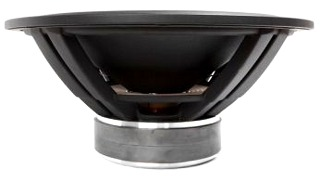open a bigger photo

recommended cabinet 1:
closed cabinet with 77 L volume
from 48/30 Hz (-3dB/-8dB)

recommended cabinet 2:
277 L volume bass reflex cabinet
with 2x HP100 reflex tube, 17 cm long.
from 24/19 Hz (-3dB/-8dB).

## SB Acoustics 15” SB42FHC75-6

order no. sb-42FHC75-6

EUR 430,50excl. VAT: € 361.76 / \$ 401.56

.

• power handling (continuous/programme) = 300 W
• resonance frequency fs = 22,5 Hz
• impedance R = 6 Ohm
• sound pressure level SPL = 93 dB (2,83V; 1m)
• DC resistance Re = 4,6 Ohm
• force factor BL = 16,2 N/A
• voice coil inductance L = 2,1 mH
• effective piston radiating area Sd = 850 cm2
• effective mechanical mass incl. air load mms = 160 g
• equivalent volume of compliance Vas = 318 l
• total Q factor Qts = 0,37 (Qms=5,4, Qes=0,4)
• voice coil diameter = 75,6 mm
• mounting diameter d = 371 mm
• overall diameter d = 417 mm
• mounting depth (not countersunk) t = 170,3 mm
PDF data sheetopen a bigger photo

## SB Acoustics 10” SB29NRX-00

order no. sb-29NRX-00

EUR 92,30excl. VAT: € 77.56 / \$ 86.09

.

• resonance frequency fs = 10,8 Hz
• effective piston radiating area Sd = 312 cm2
• effective mechanical mass incl. air load mms = 455 g
• equivalent volume of compliance Vas = 66 l
• total Q factor Qts = (Qms=10,3, Qes=)
• mounting diameter d = 249 mm
• overall diameter d = 290 mm
• mounting depth (not countersunk) t = 79,1 mm
PDF data sheetopen a bigger photo

## SB Acoustics DW50

order no. sb-DW50

EUR 5,00excl. VAT: € 4.20 / \$ 4.66

.

• effective mechanical mass incl. air load mms = 50 g
• overall diameter d = 70 mm
• mounting depth (not countersunk) t = 4,9 mm
PDF data sheetopen a bigger photo

## SB Acoustics 12” SB34NRX-00

order no. sb-34NRX-00

EUR 114,80excl. VAT: € 96.47 / \$ 107.08

.

• resonance frequency fs = 9,1 Hz
• effective piston radiating area Sd = 508 cm2
• effective mechanical mass incl. air load mms = 615 g
• equivalent volume of compliance Vas = 183 l
• total Q factor Qts = (Qms=11,7, Qes=)
• mounting diameter d = 305,18 mm
• overall diameter d = 346,2 mm
• mounting depth (not countersunk) t = 101,95 mm
PDF data sheet

## no longer soldopen a bigger photo

recommended cabinet 1:
closed cabinet with 25 L volume
from 37/23 Hz (-3dB/-8dB)

recommended cabinet 2:
100 L volume bass reflex cabinet
with HP70 reflex tube, 24 cm long.
from 17/14 Hz (-3dB/-8dB).

## SB Acoustics 10” SW26DAC76-4

order no. sb-SW26DAC76-4

EUR 259,00excl. VAT: € 217.65 / \$ 241.59

.

• power handling (continuous/programme) = 200 W
• resonance frequency fs = 22 Hz
• impedance R = 4 Ohm
• sound pressure level SPL = 86 dB (2,83V; 1m)
• DC resistance Re = 3,2 Ohm
• force factor BL = 10,8 N/A
• effective piston radiating area Sd = 312 cm2
• effective mechanical mass incl. air load mms = 134 g
• equivalent volume of compliance Vas = 54 l
• total Q factor Qts = 0,46 (Qms=4,5, Qes=0,51)
• voice coil diameter = 75,5 mm
• mounting diameter d = 244 mm
• overall diameter d = 290 mm
• mounting depth (not countersunk) t = 71,5 mm
PDF data sheet

 Tweeter Mids Woofer Passive Radiators no longer sold
The cabinet volume calculations are executed considering an external resistance of 0.3 Ohm. We are using, if no other data is known or available, the information supplied by the manufacturer.

Please note: the frequency response doesn't say much about the cut-off frequency inside the cabinet! Therefore, we mostly give you 2 examples with dimensioning considerations and an indication, how low the loudspeaker will reproduce.

Home